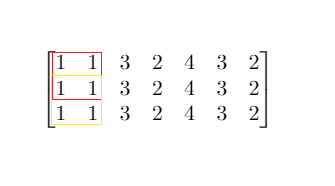## 704. 二分查找

• 你可以假设 nums 中的所有元素是不重复的
• n 将在 [1, 10000]之间
• nums 的每个元素都将在 [-9999, 9999]之间

## 153. 寻找旋转排序数组中的最小值

( 例如，数组 `[0,1,2,4,5,6,7]` 可能变为 `[4,5,6,7,0,1,2]` )。

## 154. 寻找旋转排序数组中的最小值 II

( 例如，数组 `[0,1,2,4,5,6,7]` 可能变为 `[4,5,6,7,0,1,2]` )。

• 这道题是 寻找旋转排序数组中的最小值 的延伸题目。
• 允许重复会影响算法的时间复杂度吗？会如何影响，为什么？

## 33. 搜索旋转排序数组

( 例如，数组 `[0,1,2,4,5,6,7]` 可能变为 `[4,5,6,7,0,1,2]` )。

1ms，99% 纯if判断targetmid的位置，然后选择移动lo还是hi，一开始我随便找了几组数然后就开始写，写到后面发现都是bug😂，这里画个图很方便`(nums[mid]>=nums)==(target>=nums)` 这一步很巧妙，满足这个关系就说明mid和target在同一段，不用变化，可以直接求，否则就根据mid的位置考虑如何变化

## 81. 搜索旋转排序数组 II

( 例如，数组 `[0,0,1,2,2,5,6]` 可能变为 `[2,5,6,0,0,1,2]` )。

• 这是 搜索旋转排序数组 的延伸题目，本题中的 `nums` 可能包含重复元素。
• 这会影响到程序的时间复杂度吗？会有怎样的影响，为什么？

WA哭了，好难搞，要是在工程上我肯定直接遍历了，太细节了这波

## 744. 寻找比目标字母大的最小字母

• 如果目标字母 `target = 'z'` 并且字符列表为 `letters = ['a', 'b']`，则答案返回 `'a'`

1. `letters`长度范围在`[2, 10000]`区间内。
2. `letters` 仅由小写字母组成，最少包含两个不同的字母。
3. 目标字母`target` 是一个小写字母。

## 34. 在排序数组中查找元素的第一个和最后一个位置

1ms ，99% 核心就是两次二分，分别向左和向后二分整个数组， 在相等的时候并不返回，多判断一下，左边的就控制hi向左边继续找，右边就控制lo向右边继续找，直到下一个不等于target就返回，和上面一题一样都是二分的变种

## 面试题53 - II. 0～n-1中缺失的数字

`1 <= 数组长度 <= 10000`

## 287. 寻找重复数

• 不能更改原数组（假设数组是只读的）。
• 只能使用额外的 O(1) 的空间。
• 时间复杂度小于 O(n2) 。
• 数组中只有一个重复的数字，但它可能不止重复出现一次

## 852. 山脉数组的峰顶索引

• A.length >= 3
• 存在 0 < i < A.length - 1 使得A < A < … A[i-1] < A[i] > A[i+1] > … > A[A.length - 1]

## 1095. 山脉数组中查找目标值

（这是一个 交互式问题

• `A < A < ... A[i-1] < A[i]`
• `A[i] > A[i+1] > ... > A[A.length - 1]`

• `MountainArray.get(k)` - 会返回数组中索引为`k` 的元素（下标从 0 开始）
• `MountainArray.length()` - 会返回该数组的长度

`MountainArray.get` 发起超过 `100` 次调用的提交将被视为错误答案。此外，任何试图规避判题系统的解决方案都将会导致比赛资格被取消。

• `3 <= mountain_arr.length() <= 10000`
• `0 <= target <= 10^9`
• `0 <= mountain_arr.get(index) <= 10^9`

UPDATE: 2020.7.14

## 162. 寻找峰值

liweiwei大佬的二分模板真好用！！！

## 74. 搜索二维矩阵

😔，这题wa了11次，是的，11次，可想而知我有多彩，最后写出来的解法还是如此的难看，主要就是在确定在哪一行的时候写出了好多问题，可以看到我上下的两种二分方法是不一样的，前期就揪着一种写，按照上面的板子写，结果写出了一堆bug… 以后写二分还是要注意啊，1s确定思路，代码写了3h。。。。

## 4. 寻找两个有序数组的中位数

Hard题，首先想到的是归并，但是时间复杂度不符合要求，最低要求 `O(log(m+n))`，想了好一会儿实在是想不出来（菜）然后看了评论区的解法

## 658. 找到 K 个最接近的元素

1. k 的值为正数，且总是小于给定排序数组的长度。
2. 数组不为空，且长度不超过 104
3. 数组里的每个元素与 x 的绝对值不超过 104

## 1283. 使结果不超过阈值的最小除数

• `1 <= nums.length <= 5 * 10^4`
• `1 <= nums[i] <= 10^6`
• `nums.length <= threshold <= 10^6`

## 1011. 在 D 天内送达包裹的能力

1. `1 <= D <= weights.length <= 50000`
2. `1 <= weights[i] <= 500`

## 875. 爱吃香蕉的珂珂

• `1 <= piles.length <= 10^4`
• `piles.length <= H <= 10^9`
• `1 <= piles[i] <= 10^9`

## 1292. 元素和小于等于阈值的正方形的最大边长• `1 <= m, n <= 300`
• `m == mat.length`
• `n == mat[i].length`
• `0 <= mat[i][j] <= 10000`
• `0 <= threshold <= 10^5`

## 1300. 转变数组后最接近目标值的数组和

• `1 <= arr.length <= 10^4`
• `1 <= arr[i], target <= 10^5`

## LCP 12. 小张刷题计划

• `1 <= time.length <= 10^5`
• `1 <= time[i] <= 10000`
• `1 <= m <= 1000`

## 410. 分割数组的最大值

• 1 ≤ n ≤ 1000
• 1 ≤ m ≤ min(50, n)

Hard题，但是感觉和前面的mid差不多，没啥好说的，个人感觉这题还没上面的[LCP 12. 小张刷题计划](#LCP 12-小张刷题计划) 难，不过有哥case挺恶心，算的sum会溢出，害我WA了一次，但是他结果返回的又是个int，这就很蠢

## 1482. 制作 m 束花所需的最少天数

• bloomDay.length == n
• 1 <= n <= 10^5
• 1 <= bloomDay[i] <= 10^9
• 1 <= m <= 10^6
• 1 <= k <= n

193th周赛的T3，没参加，但是在群里听群友讨论了，是个二分，刚刚具体的看了题目，发现其实是很明显的二分答案，很可惜没参加这次比赛，感觉能A3道。。。

## 378. 有序矩阵中第K小的元素

Difficulty: 中等

（直接搬运我在lc题解区写的题解

k=2，对应结果应该是5，但是我们现在mid=8，这里8和5在矩阵中小于等于它们的数量是相同的，这个时候很明显应该缩短right去逼近5，所以我们应该选取`>=`作为答案区间并记录答案，并且缩短right逼近矩阵中真实存在的值

## 441. 排列硬币

Difficulty: 简单

_n _是一个非负整数，并且在32位有符号整型的范围内。

## 174. 地下城游戏

Difficulty: 困难

 -2 (K) -3 3 -5 -10 1 10 30 -5 (P)

• 骑士的健康点数没有上限。

• 任何房间都可能对骑士的健康点数造成威胁，也可能增加骑士的健康点数，包括骑士进入的左上角房间以及公主被监禁的右下角房间。